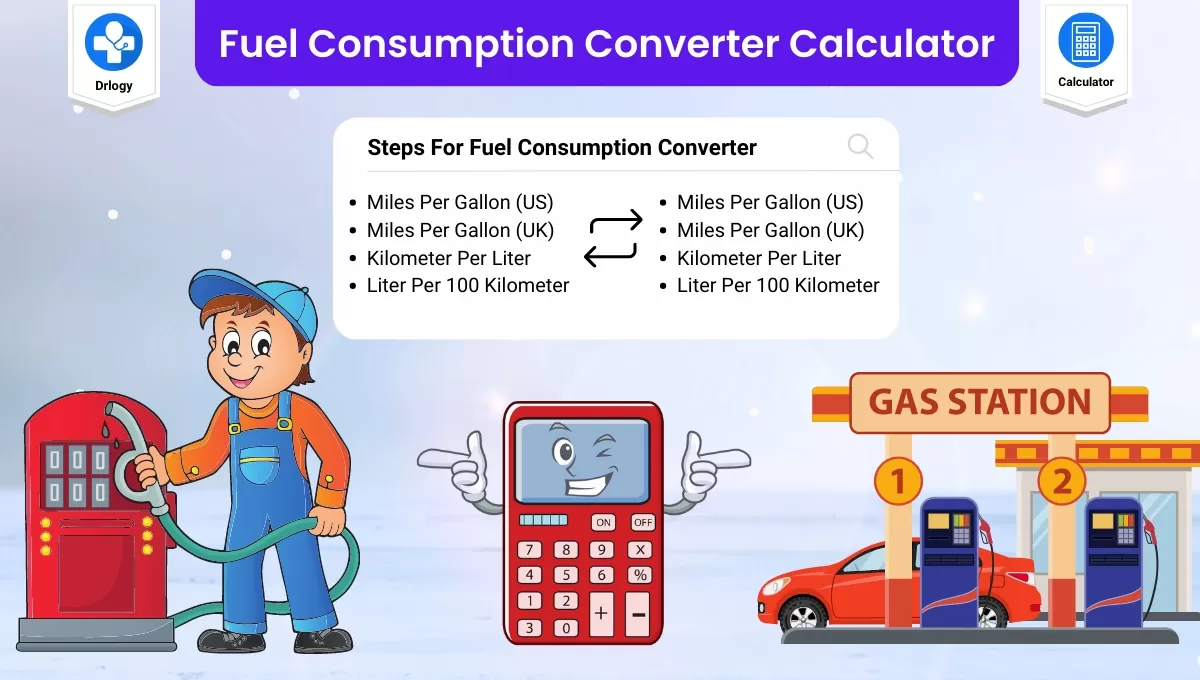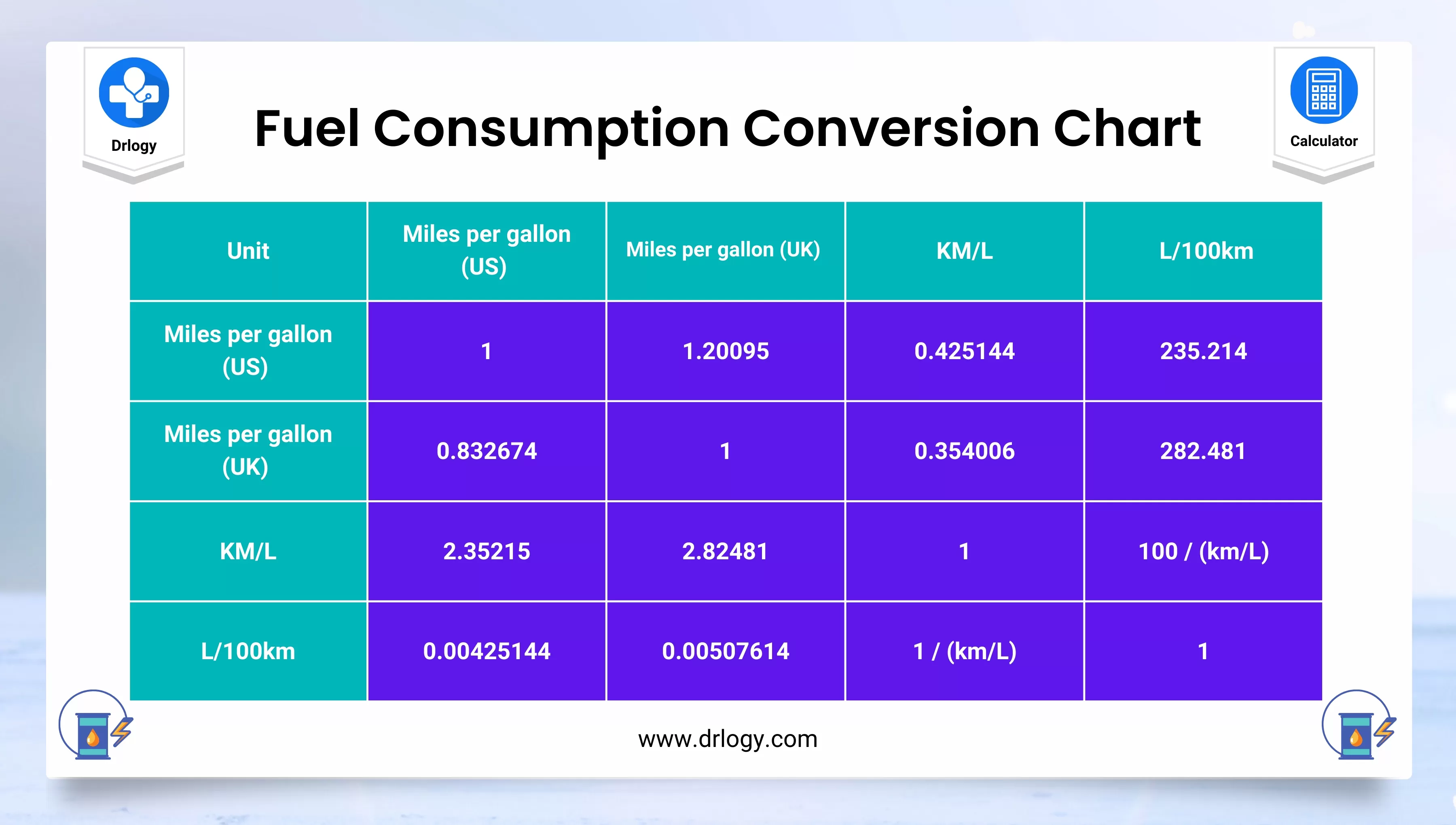• Health
• Pet
• Unit Conversion
• Medical# Fuel Economy Converter - Fuel Consumption Conversion

## Fuel Consumption Converter

Result : =
keyboard_arrow_down
=
keyboard_arrow_down

## Fuel Consumption Converter Overview

• Fuel consumption converter is a useful tool that allows you to easily convert between different units of fuel consumption.
• This tool can be helpful for anyone who wants to calculate fuel efficiency or compare the fuel consumption of different vehicles.
• With a fuel consumption converter, you can convert between common units such as miles per gallon (mpg), liters per 100 kilometers (L/100km), and kilometers per liter (km/L), among others.

## Steps For Fuel Consumption Converter

Here are the steps for using a fuel consumption converter:

1. Determine the unit of fuel consumption you would like to convert from and to. For example, you may want to convert from miles per gallon (mpg) to liters per 100 kilometers (L/100km).
2. Find a fuel consumption converter tool, either online or in a mobile app. There are many options available, so choose one that is easy to use and provides accurate conversions.
3. Input the value you would like to convert. For example, if you want to convert 30 miles per gallon to liters per 100 kilometers, input "30" in the appropriate field.
4. Select the unit of fuel consumption you are converting from. For example, if you input 30 and want to convert from miles per gallon to liters per 100 kilometers, select "mpg."
5. Select the unit of fuel consumption you are converting to. For example, if you want to convert from miles per gallon to liters per 100 kilometers, select "L/100km."
6. Review the result and confirm that it makes sense. If you are not familiar with the units of fuel consumption you are working with, do some research to ensure that the result is accurate.

By following these steps, you can easily convert between different units of fuel consumption and make informed decisions about your vehicle and fuel usage.

## Fuel Consumption Converter Chart

Here is a chart showing common units of fuel consumption and their equivalent values:

Unit Abbreviation Equivalent Value
Miles per gallon mpg 1 mpg = 0.425 km/L
Kilometers per liter km/L 1 km/L = 2.352 mpg
Liters per 100 kilometers L/100km 1 L/100km = 282.5 mpg

This chart can be used as a reference when converting between units of fuel consumption.• For example, if you have a fuel consumption value in miles per gallon and want to convert it to kilometers per liter, you can multiply the value by 0.425.
• Conversely, if you have a value in kilometers per liter and want to convert it to miles per gallon, you can multiply the value by 2.352.
• Finally, if you have a value in liters per 100 kilometers and want to convert it to miles per gallon, you can divide the value by 282.5.

## Fuel Consumption Conversion Units

There are different units used for measuring fuel consumption around the world. The two most common units are:

### Miles per gallon (MPG)

• This is a unit of measurement used mainly in the United States and the United Kingdom.
• It measures the distance traveled in miles per unit volume of fuel consumed (gallons).

### Liters per 100 kilometers (L/100km)

• This is a unit of measurement used mainly in Europe, Canada, and Australia.
• It measures the volume of fuel consumed (in liters) per unit distance traveled (in kilometers).

### Kilometers per liter (km/L)

• This is a unit of measurement used in some Asian countries, such as Japan and Thailand.
• It measures the distance traveled in kilometers per unit volume of fuel consumed (liters).

### Gallons per mile (GPM)

• This is a less common unit of measurement used in the United States.
• It measures the volume of fuel consumed (in gallons) per unit distance traveled (in miles).

### Liters per kilometer (L/km)

• This is a less common unit of measurement used in some European countries.
• It measures the volume of fuel consumed (in liters) per unit distance traveled (in kilometers).

When using a fuel consumption converter, it's important to ensure that you are converting between the same units of measurement.

## Fuel Consumption Converter Formula

There are different units for measuring fuel consumption, but the two most common ones are:

• Miles per gallon (MPG): this is the distance travelled in miles per unit volume of fuel consumed (gallons).
• Liters per 100 kilometers (L/100km): this is the volume of fuel consumed (in liters) per unit distance travelled (in kilometers).

To convert from MPG to L/100km, you can use the following formula:

L/100km = 235.215 / MPG

To convert from L/100km to MPG, you can use the following formula:

MPG = 235.215 / L/100km

Note that these formulas are approximate, as fuel consumption can vary depending on driving conditions, vehicle type, and other factors.

## Fuel Consumption Converter Table

Here is a table showing a conversion chart for fuel consumption:

Miles per Gallon (mpg) Kilometers per Liter (km/L) Liters per 100 Kilometers (L/100km)
5 0.212 47.04
10 0.425 23.52
15 0.637 15.68
20 0.850 11.76
25 1.063 9.41
30 1.275 7.84
35 1.488 6.74
40 1.700 5.88
45 1.913 5.22
50 2.125 4.71

To use this table, find the value you want to convert in the column corresponding to the unit you are converting from. Then, locate the corresponding value in the column for the unit you are converting to according to above table.

## Benefits of Fuel Consumption Converter

There are several benefits of using a fuel consumption converter, including:

1. Comparison: By converting fuel consumption units, you can compare the fuel efficiency of different vehicles, engines, or modes of transportation. This can help you make informed decisions when purchasing a vehicle or choosing a mode of transportation.
2. Cost savings: By knowing the fuel consumption of your vehicle or the vehicle you plan to purchase, you can estimate your fuel costs and plan your budget accordingly. You can also compare fuel prices and choose the most cost-effective fuel for your vehicle.
3. Environmental impact: Fuel consumption is directly related to greenhouse gas emissions and air pollution. By using a fuel consumption converter, you can estimate the environmental impact of your vehicle and make choices that reduce your carbon footprint.
4. Accuracy: Fuel consumption converters use standardized units of measurement and accurate formulas to convert fuel consumption values. This ensures that you have accurate information that can help you make informed decisions about fuel efficiency and cost savings.

Summary

Overall, using a fuel consumption converter can help you make more informed decisions about your vehicle, fuel consumption, and environmental impact. Check more unit conversion calculators like this to solve your daily problems on Drlogy Calculator to get exact solution.

Reference

• Fuel Consumption - an overview .
• Fuel consumption - Energy Education .
favorite_border 1526 Likes

## Fuel Consumption Converter FAQ

### What is a good fuel economy?

A good fuel economy is a relative term and can depend on several factors, such as the type of vehicle, the driving conditions, and the fuel type. Here is a table showing the fuel economy ratings for cars based on EPA ratings in the United States:

Fuel Economy Rating (MPG) Classification
Less than 15 Poor
15 - 20 Fair
21 - 25 Good
26 - 30 Very Good
31 - 40 Excellent
41 and above Outstanding

Note that these ratings are based on standardized tests and may not accurately reflect real-world driving conditions or individual driving habits. Additionally, fuel economy can vary based on the type of vehicle and the fuel type used. Therefore, it's important to consider other factors, such as the cost of fuel and the environmental impact, when evaluating the fuel economy of a vehicle.

### How do you convert fuel consumption?

To convert fuel consumption, you need to know the value in the original unit of measurement and the conversion factor for the unit you want to convert to. Here are the formulas for converting fuel consumption between miles per gallon (MPG) and liters per 100 kilometers (L/100km):

• To convert MPG to L/100km:
1. Multiply the MPG value by 235.214.
2. Divide the result by 100.

Formula: L/100km = 235.214 / MPG

• To convert L/100km to MPG:
1. Divide the value in L/100km by 235.214.
2. Divide the result into 1.

Formula: MPG = 1 / (L/100km / 235.214)

• Here's an example conversion from MPG to L/100km:

Convert 30 MPG to L/100km

L/100km = 235.214 / 30 L/100km = 7.84

Therefore, 30 MPG is equivalent to 7.84 L/100km.

• Here's an example conversion from L/100km to MPG:

Convert 8 L/100km to MPG

MPG = 1 / (8 / 235.214) MPG = 29.4

Therefore, 8 L/100km is equivalent to 29.4 MPG.

### How to convert L 100km to kmpl?

To convert liters per 100 kilometers (L/100km) to kilometers per liter (km/L or kmpl), you can use the following formula:

km/L = 100 / L/100km / 100

Here's an example conversion:

Convert 10 L/100km to kmpl:

km/L = 100 / 10 / 100 km/L = 0.1 kmpl

Therefore, 10 L/100km is equivalent to 0.1 kmpl. Note that this conversion factor is the reciprocal of the one used to convert kmpl to L/100km, which is:

L/100km = 100 / km/L / 100

Using this formula, you can convert km/L to L/100km by dividing 100 by the value in km/L and then multiplying by 100.

View More Health

## Medical Calculator

View More Medical

View More Pet

## Unit Conversion Calculator

View More Unit Conversion

## Financial Calculator

View More Financial
• Home >
• Calculator >
• Fuel Economy Converter - Fuel Consumption Conversion
Drlogy®
The Power To Health